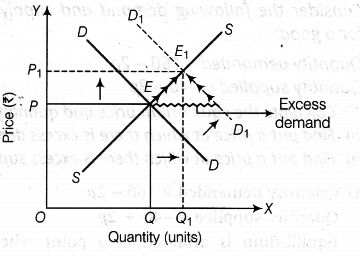# How is the equilibrium price

How is the equilibrium price and equilibrium quantity of a normal commodity affected by an increase in the income of the buyers? Explain with the help of a diagram.

When income of the consumer rises, demand curve will shift to the right, supply curve remains unaffected. However, when consumers are willing to pay higher price for the same quantity (because of increase in their income), price would tend to rise.
Consequently, quantity supplied by the producers would tend to rise.Thus, increase in demand and the consequent shift in demand curve to the right impacts producer’s decisions by way of extension of supply in response to increase in price. Finally, you would end up in a situation when equilibrium price as well as equilibrium quantity tends to rise, in response to increase in demand.
Here, OP = Initial equilibrium price
OQ = Initial equilibrium quantity
{{OP}_{1}} = New equilibrium price .
{{OQ}_{1}} = New equilibrium quantity